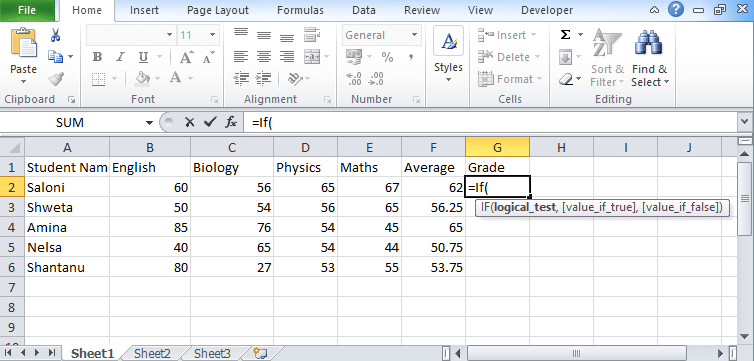To advertise with us contact on Whatsapp: +923041280395 For guest post email at: [email protected]

# Excel Logical Test Using Multiple If Statements [OR/AND]To test certain conditions, people use If statement. The If() function is used to perform logical tests and to evaluate conditions that will show two outcomes. This excel if statement is used to analyze a condition that will show two outcomes. Basically, excel logical test is used to evaluate the condition whether it is true or false.

## Excel Logical Test

If statement is mainly used to test any condition that will give you more than two outcomes. If you are using a single If then it will give you two outcomes. For more than two outcomes you need to use If statement inside another If statement.

## Multiple If Statement in Excel for Three Outcomes

For multiple situations, you have to apply multiple If statement to get more than two outcomes. This means that you are creating if function within another if function.

### Syntax

If(logical_test, [value_if_True], [Value_if_false])

### How to apply

• Lets take a data set for calculating commission on sales target.

You have conditions:

Less than \$300: Receive 7% commission

Sales btw \$300 to \$600: Receive 12.5% commission

Sales more than \$600: Receive 16% commission

Now to find commission, we have to apply If statement. You must use nested if to calculate commission of all salesperson.

• To calculate commission, the formula is “=IF(B2<300,B2*7%, IF(B2<600,B2*12.5%,B2*16%))
• After finding the commission, you need to press enter key and then calculate the commission.
• Using Autofill feature, you can calculate the commission of remaining person.

Hence, in this situation, if statement will check whether the value in B2 is greater or less than \$300. If it is so, then the commission is 7%. If B2 is in between \$300 to \$700 then the commission will be calculated at 12.5% and if the figure is more than \$700, then the commission will be calculated via 16% rate.

## Multiple If Statement in Excel for Four Outcomes

In these four outcomes, you require three different conditions. Excel If statement multiple conditions range tests a single condition and returns an outcome.

### Syntax

=IF(Condition A, Output Y, IF (Condition B, Output Z, IF (Condition C, Output R, Output S))))

In this formula, we have three conditions that will show 4 outcomes.

We start with, testing If statement A is true or not. If it is true then it will return output B

But if the condition is False then testing of B condition will start

If condition B is true, then the output will be Z and if it is false, the testing of condition C will begin.

If condition C is true then it returns R else S.

Let us take the situation and conditions

• Average>=60 : Credit
• Average>=50 : Pass
• Average<50 : Fail

Syntax to find the grade is

=If(F2>=70,”Distinction”,IF(F2>=60,”CREDIT”,IF(G2>50,”PASS”,”FAIL”)))

### How to apply

• Take a dataset of students.
• Along with the name, type the numbers that they get in each subject.
• Calculate the average of every student.
• On the basis of average marks, calculate the grade of the student.
• For calculating the grade, you have to take the above condition.
• In the grade section, write the formula for grade and press enter.
• After you get the outcome in cell G2, drag the cell border below.

This is how you will be going to perform excel logical test on multiple conditions to get different outcomes.